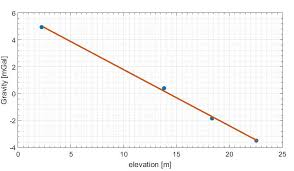## How to Calculate and Solve for Bouguer Correction | GravityThe image above represents bourger correction.

To compute for bourger correction, three essential parameter are needed and these parameters are Gravitational Constant (G), Density of the Material (ρ) and Height at Measured Point above Sea Level (h).

The formula for calculating bourger correction:

δgB = 2πGρh

Where:

δgB = Bouguer Correction
G = Gravitational Constant
ρ = Density of the Material
h = Height at Measured Point above Sea Level

Let’s solve an example;
Find the bourger correction when the gravitational constant is 6.67E-11, the density of the material is 24 and the height at measured point above sea level is 18.

This implies that;

G = Gravitational Constant = 6.67E-11
ρ = Density of the Material = 24
h = Height at Measured Point above Sea Level = 18

δgB = 2πGρh
δgB = 2π(6.67e-11)(24)(18)
δgB = 2π(2.88144e-8)
δgB = 1.81e-7

Therefore, the bourger correction is 1.81e-7 mGal.